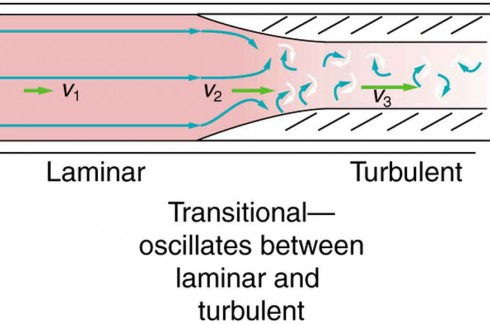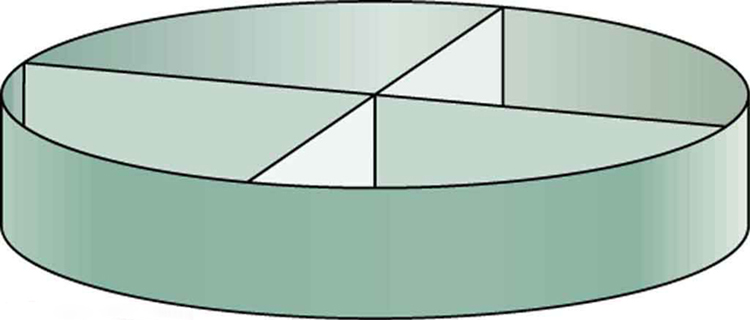## The Onset of Turbulence

### Learning Objectives

By the end of this section, you will be able to:

• Calculate Reynolds number.
• Use the Reynolds number for a system to determine whether it is laminar or turbulent.

Sometimes we can predict if flow will be laminar or turbulent. We know that flow in a very smooth tube or around a smooth, streamlined object will be laminar at low velocity. We also know that at high velocity, even flow in a smooth tube or around a smooth object will experience turbulence. In between, it is more difficult to predict. In fact, at intermediate velocities, flow may oscillate back and forth indefinitely between laminar and turbulent.

An occlusion, or narrowing, of an artery, such as shown in Figure 1, is likely to cause turbulence because of the irregularity of the blockage, as well as the complexity of blood as a fluid. Turbulence in the circulatory system is noisy and can sometimes be detected with a stethoscope, such as when measuring diastolic pressure in the upper arm’s partially collapsed brachial artery. These turbulent sounds, at the onset of blood flow when the cuff pressure becomes sufficiently small, are called Korotkoff sounds. Aneurysms, or ballooning of arteries, create significant turbulence and can sometimes be detected with a stethoscope. Heart murmurs, consistent with their name, are sounds produced by turbulent flow around damaged and insufficiently closed heart valves. Ultrasound can also be used to detect turbulence as a medical indicator in a process analogous to Doppler-shift radar used to detect storms.Figure 1. Flow is laminar in the large part of this blood vessel and turbulent in the part narrowed by plaque, where velocity is high. In the transition region, the flow can oscillate chaotically between laminar and turbulent flow.

An indicator called the Reynolds number NR can reveal whether flow is laminar or turbulent. For flow in a tube of uniform diameter, the Reynolds number is defined as

${N}_{\text{R}}=\frac{2\rho {vr}}{\eta}\text{(flow in tube)}$,

where ρ is the fluid density, v its speed, η its viscosity, and r the tube radius. The Reynolds number is a unitless quantity. Experiments have revealed that NR is related to the onset of turbulence. For NR below about 2000, flow is laminar. For NR above about 3000, flow is turbulent. For values of NR between about 2000 and 3000, flow is unstable—that is, it can be laminar, but small obstructions and surface roughness can make it turbulent, and it may oscillate randomly between being laminar and turbulent. The blood flow through most of the body is a quiet, laminar flow. The exception is in the aorta, where the speed of the blood flow rises above a critical value of 35 m/s and becomes turbulent.

### Example 1. Is This Flow Laminar or Turbulent?

Calculate the Reynolds number for flow in the needle considered in Example 2 from Viscosity and Laminar Flow to verify the assumption that the flow is laminar. Assume that the density of the saline solution is 1025 kg/m3.

#### Strategy

We have all of the information needed, except the fluid speed v, which can be calculated from $\overline{v}=Q/A=1.70\text{ m/s}\\$ (verification of this is in this chapter’s Problems and Exercises).

#### Solution

Entering the known values into ${N}_{\text{R}}=\frac{2\rho \text{vr}}{\eta }\\$ gives

$\begin{array}{lll}{N}_{\text{R}}& =& \frac{2\rho \text{vr}}{\eta }\\ & =& \frac{2\left({\text{1025 kg/m}}^{3}\right)\left(\text{1.70 m/s}\right)\left(0.150\times {\text{10}}^{-3}\text{m}\right)}{1\text{.}\text{00}\times {\text{10}}^{-3}\text{N}\cdot {\text{s/m}}^{2}}\\ & =& 523\end{array}\\$.

#### Discussion

Since NR is well below 2000, the flow should indeed be laminar.

### Take-Home Experiment: Inhalation

Under the conditions of normal activity, an adult inhales about 1 L of air during each inhalation. With the aid of a watch, determine the time for one of your own inhalations by timing several breaths and dividing the total length by the number of breaths. Calculate the average flow rate Q of air traveling through the trachea during each inhalation.

The topic of chaos has become quite popular over the last few decades. A system is defined to be chaotic when its behavior is so sensitive to some factor that it is extremely difficult to predict. The field of chaos is the study of chaotic behavior. A good example of chaotic behavior is the flow of a fluid with a Reynolds number between 2000 and 3000. Whether or not the flow is turbulent is difficult, but not impossible, to predict—the difficulty lies in the extremely sensitive dependence on factors like roughness and obstructions on the nature of the flow. A tiny variation in one factor has an exaggerated (or nonlinear) effect on the flow. Phenomena as disparate as turbulence, the orbit of Pluto, and the onset of irregular heartbeats are chaotic and can be analyzed with similar techniques.

## Section Summary

• The Reynolds number NR can reveal whether flow is laminar or turbulent. It is
${N}_{\text{R}}=\frac{2\rho{vr}}{\eta}\\$.
• For NR below about 2000, flow is laminar. For NR above about 3000, flow is turbulent. For values of NR between 2000 and 3000, it may be either or both.

### Conceptual Questions

1. Doppler ultrasound can be used to measure the speed of blood in the body. If there is a partial constriction of an artery, where would you expect blood speed to be greatest, at or nearby the constriction? What are the two distinct causes of higher resistance in the constriction?

2. Sink drains often have a device such as that shown in Figure 2 to help speed the flow of water. How does this work?Figure 2. You will find devices such as this in many drains. They significantly increase flow rate.

3. Some ceiling fans have decorative wicker reeds on their blades. Discuss whether these fans are as quiet and efficient as those with smooth blades.

### Problems & Exercises

1. Verify that the flow of oil is laminar (barely) for an oil gusher that shoots crude oil 25.0 m into the air through a pipe with a 0.100-m diameter. The vertical pipe is 50 m long. Take the density of the oil to be 900 kg/m3 and its viscosity to be 1.00 (N/m2)⋅s (or 1.00 P ⋅ s).

2. Show that the Reynolds number NR is unitless by substituting units for all the quantities in its definition and cancelling.

3. Calculate the Reynolds numbers for the flow of water through (a) a nozzle with a radius of 0.250 cm and (b) a garden hose with a radius of 0.900 cm, when the nozzle is attached to the hose. The flow rate through hose and nozzle is 0.500 L/s. Can the flow in either possibly be laminar?

4. A fire hose has an inside diameter of 6.40 cm. Suppose such a hose carries a flow of 40.0 L/s starting at a gauge pressure of 1.62 × 106 N/m2. The hose goes 10.0 m up a ladder to a nozzle having an inside diameter of 3.00 cm. Calculate the Reynolds numbers for flow in the fire hose and nozzle to show that the flow in each must be turbulent.

5. Concrete is pumped from a cement mixer to the place it is being laid, instead of being carried in wheelbarrows. The flow rate is 200.0 L/min through a 50.0-m-long, 8.00-cm-diameter hose, and the pressure at the pump is 8.00 × 106 N/m2. Verify that the flow of concrete is laminar taking concrete’s viscosity to be 48.0 (N/m2) · s, and given its density is 2300 kg/m3.

6. At what flow rate might turbulence begin to develop in a water main with a 0.200-m diameter? Assume a 20ºC temperature.

7. What is the greatest average speed of blood flow at 37ºC in an artery of radius 2.00 mm if the flow is to remain laminar? What is the corresponding flow rate? Take the density of blood to be 1025 kg/m3.

8. In Take-Home Experiment: Inhalation, we measured the average flow rate of air traveling through the trachea during each inhalation. Now calculate the average air speed in meters per second through your trachea during each inhalation. The radius of the trachea in adult humans is approximately 10-2 m. From the data above, calculate the Reynolds number for the air flow in the trachea during inhalation. Do you expect the air flow to be laminar or turbulent?

9. Gasoline is piped underground from refineries to major users. The flow rate is 3.00 × 10-2 m3/s (about 500 gal/min), the viscosity of gasoline is 1.00 × 10-3 (N/m2) ⋅ s, and its density is 680 kg/m3. (a) What minimum diameter must the pipe have if the Reynolds number is to be less than 2000? (b) What pressure difference must be maintained along each kilometer of the pipe to maintain this flow rate?

10. Assuming that blood is an ideal fluid, calculate the critical flow rate at which turbulence is a certainty in the aorta. Take the diameter of the aorta to be 2.50 cm. (Turbulence will actually occur at lower average flow rates, because blood is not an ideal fluid. Furthermore, since blood flow pulses, turbulence may occur during only the high-velocity part of each heartbeat.)

11. Unreasonable Results A fairly large garden hose has an internal radius of 0.600 cm and a length of 23.0 m. The nozzleless horizontal hose is attached to a faucet, and it delivers 50.0 L/s. (a) What water pressure is supplied by the faucet? (b) What is unreasonable about this pressure? (c) What is unreasonable about the premise? (d) What is the Reynolds number for the given flow? (Take the viscosity of water as 1.005 × 10-3 (N/m2⋅ s.)

## Glossary

Reynolds number:
a dimensionless parameter that can reveal whether a particular flow is laminar or turbulent

### Selected Solutions to Problems & Exercises

1. N= 1.99 × 102<2000

3. (a) nozzle: 1.27 × 105, not laminar (b) hose: 3.51 × 104, not laminar.

5. 2.54 << 2000, laminar.

7. 1.02 m/s, 1.28 × 10-2 L/s

9. (a) ≥ 13.0 m (b) 2.68 × 10-6 N/m2

11. (a) 23.7 atm or 344 lb/in2

(b) The pressure is much too high.

(c) The assumed flow rate is very high for a garden hose.

(d) 5.27 × 106 > > 3000, turbulent, contrary to the assumption of laminar flow when using this equation.